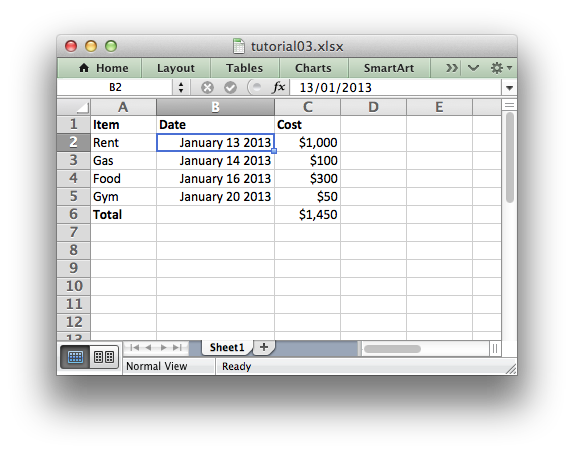libxlsxwriter
Tutorial 3: Writing different types of data to the XLSX File

In the previous section we created a simple spreadsheet with formatting using C and the `libxlsxwriter` module.

This time let's extend the data we want to write to include some dates:

Item Date Cost
Rent 2013-01-13 1000
Gas 2013-01-14 100
Food 2013-01-16 300
Gym 2013-01-20 50

The corresponding spreadsheet will look like this:The differences here are that we have added a Date column with formatting and made that column a little wider to accommodate the dates.

To do this we can extend our program as follows:

#include "xlsxwriter.h"
/* Some data we want to write to the worksheet. */
struct expense {
char item;
int cost;
lxw_datetime datetime;
};
struct expense expenses[] = {
{"Rent", 1000, { .year = 2013, .month = 1, .day = 13 } },
{"Gas", 100, { .year = 2013, .month = 1, .day = 14 } },
{"Food", 300, { .year = 2013, .month = 1, .day = 16 } },
{"Gym", 50, { .year = 2013, .month = 1, .day = 20 } },
};
int main() {
/* Create a workbook and add a worksheet. */
lxw_workbook *workbook = workbook_new("tutorial03.xlsx");
int row = 0;
int col = 0;
int i;
/* Add a bold format to use to highlight cells. */
/* Add a number format for cells with money. */
format_set_num_format(money, "\$#,##0");
/* Add an Excel date format. */
format_set_num_format(date_format, "mmmm d yyyy");
/* Adjust the column width. */
worksheet_set_column(worksheet, 0, 0, 15, NULL);
/* Write some data header. */
worksheet_write_string(worksheet, row, col, "Item", bold);
worksheet_write_string(worksheet, row, col + 1, "Cost", bold);
/* Iterate over the data and write it out element by element. */
for (i = 0; i < 4; i++) {
/* Write from the first cell below the headers. */
row = i + 1;
worksheet_write_string (worksheet, row, col, expenses[i].item, NULL);
worksheet_write_datetime(worksheet, row, col + 1, &expenses[i].datetime, date_format);
worksheet_write_number (worksheet, row, col + 2, expenses[i].cost, money);
}
/* Write a total using a formula. */
worksheet_write_string (worksheet, row + 1, col, "Total", bold);
worksheet_write_formula(worksheet, row + 1, col + 2, "=SUM(C2:C5)", money);
/* Save the workbook and free any allocated memory. */
return workbook_close(workbook);
}

The main difference between this and the previous program is that we have added a new Format object for dates and we have additional handling for the date information.

We have also extended the data that we are going to write to include a lxw_datetime struct to hold the date information. We will see how that is used shortly.

struct expense {
char item;
int cost;
lxw_datetime datetime;
};
struct expense expenses[] = {
{"Rent", 1000, { .year = 2013, .month = 1, .day = 13 } },
{"Gas", 100, { .year = 2013, .month = 1, .day = 14 } },
{"Food", 300, { .year = 2013, .month = 1, .day = 16 } },
{"Gym", 50, { .year = 2013, .month = 1, .day = 20 } },
};

Excel treats different types of input data, such as strings and numbers, differently although it generally does it transparently to the user. Libxlsxwriter tries to emulate this in the `worksheet_write*()` functions by mapping C data types to types that Excel supports.

In this version of our program we have used some of these functions for different types of data:

worksheet_write_string (worksheet, row, col, expenses[i].item, NULL);
worksheet_write_datetime(worksheet, row, col + 1, &expenses[i].datetime, date_format);
worksheet_write_number (worksheet, row, col + 2, expenses[i].cost, money);

The handling of dates is also new to our program.

Dates and times in Excel are floating point numbers that have a number format applied to display them in the correct format. Libxlsxwriter provides the worksheet_write_datetime() and the lxw_datetime struct help to convert dates and times into Excel date and time numbers.

The worksheet_write_datetime() function converts the lxw_datetime struct to a number that represents an Excel date but we also need to add the number format to ensure that Excel displays it as as date:

format_set_num_format(date_format, "mmmm d yyyy");

Date handling is explained in more detail in Working with Dates and Times.

That completes the tutorial section.

In the next sections we will look at the API in more detail starting with workbook.

Next: The Workbook object

workbook_close
lxw_error workbook_close(lxw_workbook *workbook)
Close the Workbook object and write the XLSX file.
worksheet_write_formula
lxw_error worksheet_write_formula(lxw_worksheet *worksheet, lxw_row_t row, lxw_col_t col, const char *formula, lxw_format *format)
Write a formula to a worksheet cell.
workbook_new
lxw_workbook * workbook_new(const char *filename)
Create a new workbook object.
format_set_bold
void format_set_bold(lxw_format *format)
Turn on bold for the format font.
format_set_num_format
void format_set_num_format(lxw_format *format, const char *num_format)
Set the number format for a cell.
lxw_worksheet
Struct to represent an Excel worksheet.
Definition: worksheet.h:2107
lxw_format
Struct to represent the formatting properties of an Excel format.
Definition: format.h:358
worksheet_write_datetime
lxw_error worksheet_write_datetime(lxw_worksheet *worksheet, lxw_row_t row, lxw_col_t col, lxw_datetime *datetime, lxw_format *format)
Write a date or time to a worksheet cell.
lxw_datetime
Struct to represent a date and time in Excel.
Definition: common.h:155
lxw_workbook
Struct to represent an Excel workbook.
Definition: workbook.h:292
worksheet_write_string
lxw_error worksheet_write_string(lxw_worksheet *worksheet, lxw_row_t row, lxw_col_t col, const char *string, lxw_format *format)
Write a string to a worksheet cell.
worksheet_write_number
lxw_error worksheet_write_number(lxw_worksheet *worksheet, lxw_row_t row, lxw_col_t col, double number, lxw_format *format)
Write a number to a worksheet cell.
worksheet_set_column
lxw_error worksheet_set_column(lxw_worksheet *worksheet, lxw_col_t first_col, lxw_col_t last_col, double width, lxw_format *format)
Set the properties for one or more columns of cells.